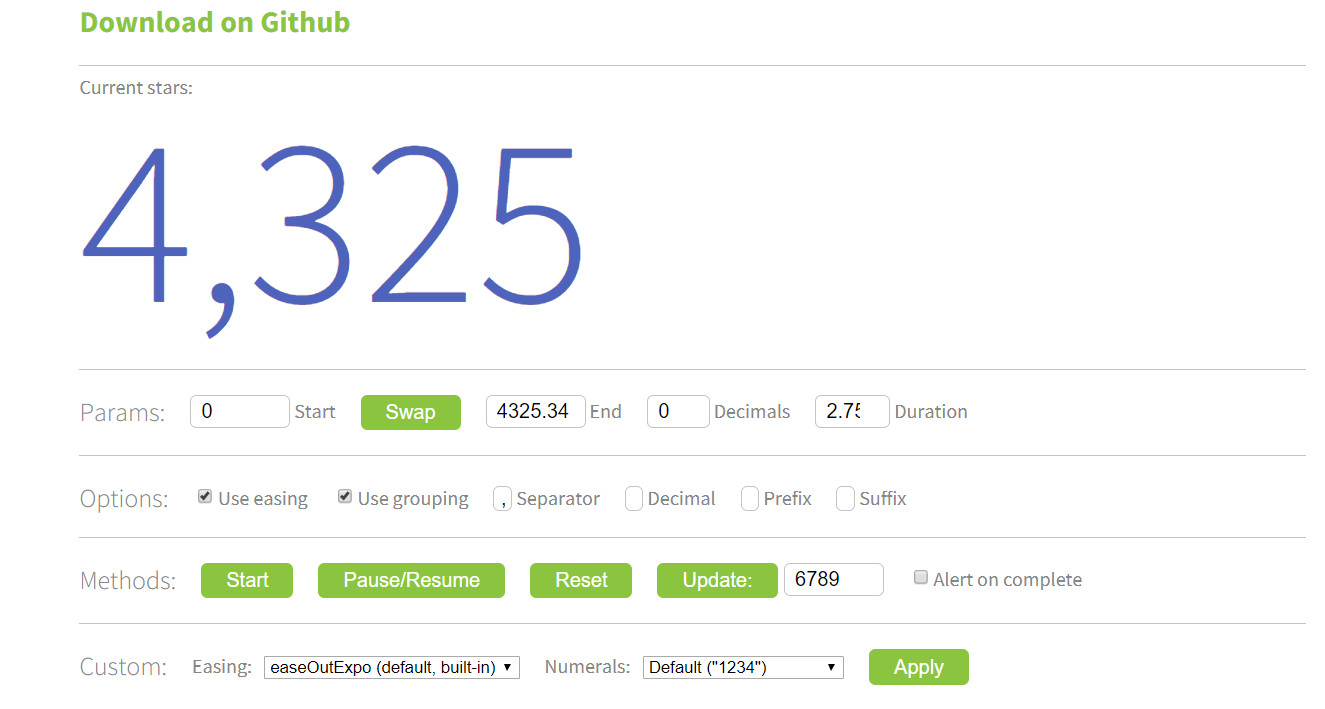# countUp.js在react中的应用

demo在查看CountUp.js工具demo

github在countUp的GitHub

yarn add react-countup

npm install react-countup --saveimport React from 'react';
import { render } from 'react-dom';
import CountUp from 'react-countup';

render(
<CountUp start={0} end={160526} />,
document.getElementById('root')
);


• start: number

• end: number

• duration: number

• decimals: number

• useEasing: boolean

• useGrouping: boolean

• separator: string

• decimal: string

• prefix: string

• suffix: string

• className: string

span元素的CSS类名

• redraw: boolean

• onComplete: function

• onStart: function

• easingFn: function

Easing function，一般用不到

• formattingFn: function

import React from 'react';
import { render } from 'react-dom';
import CountUp from 'react-countup';

const onComplete = () => {
console.log('Completed! ?');
};

const onStart = () => {
console.log('Started! ?');
};

render(
<CountUp
className="account-balance"
start={160527.0127}
end={-875.0319}
duration={2.75}
useEasing={true}
useGrouping={true}
separator=" "
decimals={4}
decimal=","
prefix="EUR "
suffix=" left"
onComplete={onComplete}
onStart={onStart}
/>,
document.getElementById('root'),
);


import CountUp from 'react-countup';

...
<CountUp
start={0}
end={bls}
duration={2.75}
useEasing
useGrouping
separator=","
suffix="份"
/>


import CountUp from 'react-countup';

...
const countUpProps = {
start: 0,
duration: 2.75,
useEasing: true,
useGrouping: true,
separator: ',',
};
<CountUp
end={bls}
suffix="份"
{...countUpProps}
/>